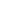Skip To Content

# University of South FloridaIntroduction to Numerical Methods - Part 2 of 2 is a Course

## Introduction to Numerical Methods - Part 2 of 2

Self-paced

Open

Ongoing

6 hours

See description

Self-paced

None

### Description

This course is the second in a two-part series that introduces numerical methods. Numerical methods are techniques to approximate mathematical procedures (e.g., integrals). Approximations are needed because we either cannot solve the procedure analytically (e.g., the standard normal cumulative distribution function) or because the analytical method is intractable (e.g., solving a set of a thousand simultaneous linear equations for a thousand unknowns).

To be prepared for this course, students should have a passing grade introductory physics, integral calculus, differential calculus, and ordinary differential equations.

### Objectives

By end of this course, participants will be able to apply the numerical methods for the following mathematical procedures and topics: simultaneous linear equations, interpolation, regression, integration, and ordinary differential equations. Additionally, they will be able to calculate errors and implement their relationship to the accuracy of the numerical solutions.

Target Audience: STEM juniors in college.

Course is offered by the University of South Florida.

### Course Instructors#### Autar Kaw

Instructor

Autar Kaw is a professor of mechanical engineering at the University of South Florida. He is a recipient of the 2012 U.S. Professor of the Year Award. He received his Ph.D. in 1987 in Engineering Mechanics from Clemson University, the same year he joined the University of South Florida. Professor Kaw's main scholarly interests are in engineering education research and open courseware development.